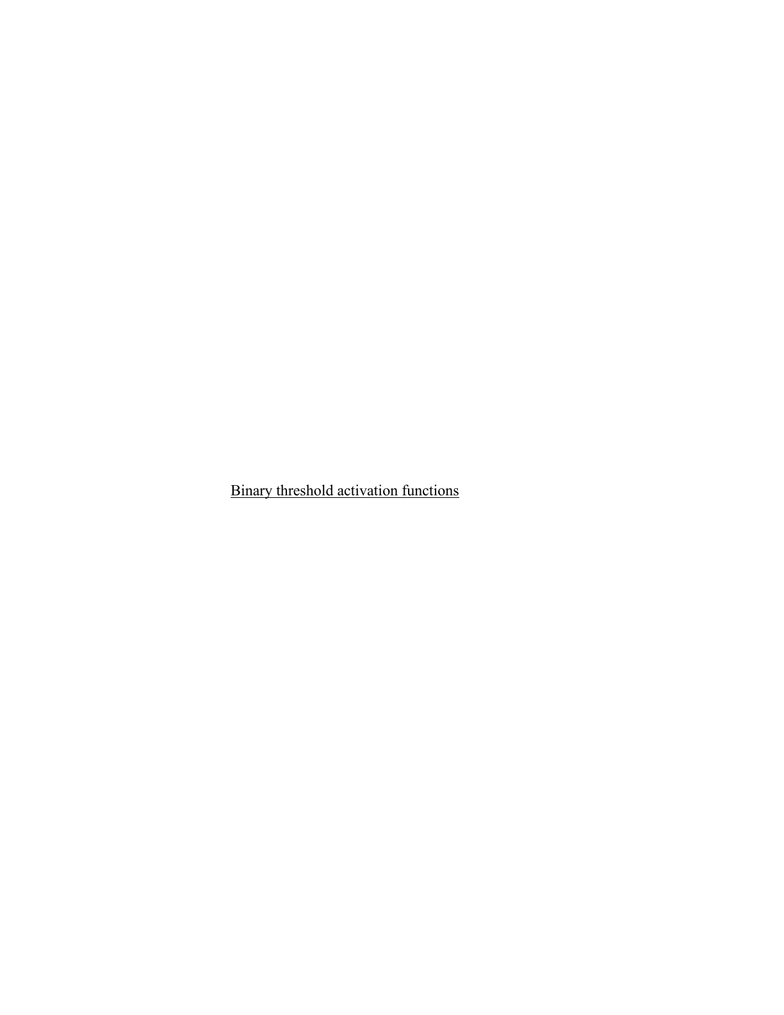Textbook Notes (280,000)
US (110,000)
UD (1,000)
CGSC (70)
CGSC170 (20)
Chapter 8.2 pt 2

# CGSC170 Chapter 8.2 pt 2:

Department
Cognitive Science
Course Code
CGSC170
Professor
Kaja Jasinka
Chapter
8.2 pt 2

This preview shows half of the first page. to view the full 3 pages of the document.Connection to Neural NEtworks
Network units in the domain and range connection can represent binary Boolean
functions.
First they must represent Boolean functions using numbers
Need numbers for input/output values
Ex- True= 1 and False= 0
If this only produces 1 or 0 as inputs and outputs than it is a
Boolean Function
If there are 2 inputs than it is binary Boolean
3 inputs= ternary Boolean
Designing Networks to take 0 and 1 as outputs
Binary threshold activation functions
These functions output 0 until the threshold is reached
Then it outputs 1
THe weights and the threshold must be set in a way that replicates the
truth table for a Boolean Function to represent this function
There are “x”-nary Boolean function for every natural number “x”
The only one scientists focus on is the Unary function
NOT
NOT A is true if A is false
NOT A is false if A is true
Individual neuron-like units can achieve a lot
A single unit can represent basic Boolean Functions